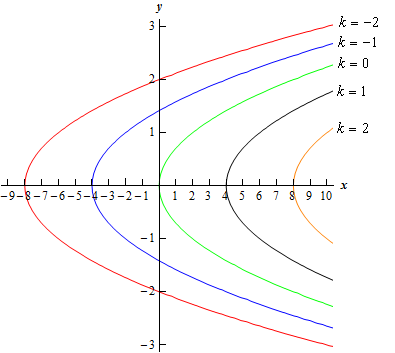Paul's Online Notes
Home / Calculus III / 3-Dimensional Space / Functions of Several Variables
Show Mobile Notice Show All Notes Hide All Notes
Mobile Notice
You appear to be on a device with a "narrow" screen width (i.e. you are probably on a mobile phone). Due to the nature of the mathematics on this site it is best views in landscape mode. If your device is not in landscape mode many of the equations will run off the side of your device (should be able to scroll to see them) and some of the menu items will be cut off due to the narrow screen width.

### Section 12.5 : Functions of Several Variables

6. Identify and sketch the level curves (or contours) for the following function.

$4z + 2{y^2} - x = 0$

Show All Steps Hide All Steps

Start Solution

We know that level curves or contours are given by setting $$z = k$$. Doing this in our equation gives,

$4k + 2{y^2} - x = 0$ Show Step 2

A quick rewrite of the equation from the previous step gives us,

$x = 2{y^2} + 4k$

So, the level curves for this function will be parabolas opening to the right and starting at 4$$k$$.

Note as well that there will be no restrictions on the values of $$k$$ that we can use, as there sometimes are.

Show Step 3

Below is a sketch of some level curves for some values of $$k$$ for this function.